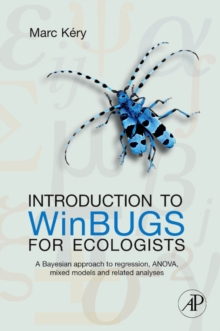Supporting your high street Find out how »
• My Account# Introduction to WinBUGS for Ecologists : Bayesian Approach to Regression, ANOVA, Mixed Models and Related Analyses Paperback / softback

#### Description

Introduction to WinBUGS for Ecologists introduces applied Bayesian modeling to ecologists using the highly acclaimed, free WinBUGS software.

It offers an understanding of statistical models as abstract representations of the various processes that give rise to a data set.

Such an understanding is basic to the development of inference models tailored to specific sampling and ecological scenarios.

The book begins by presenting the advantages of a Bayesian approach to statistics and introducing the WinBUGS software.

It reviews the four most common statistical distributions: the normal, the uniform, the binomial, and the Poisson.

It describes the two different kinds of analysis of variance (ANOVA): one-way and two- or multiway.

It looks at the general linear model, or ANCOVA, in R and WinBUGS.

It introduces generalized linear model (GLM), i.e., the extension of the normal linear model to allow error distributions other than the normal.

The GLM is then extended contain additional sources of random variation to become a generalized linear mixed model (GLMM) for a Poisson example and for a binomial example.

The final two chapters showcase two fairly novel and nonstandard versions of a GLMM.

The first is the site-occupancy model for species distributions; the second is the binomial (or N-) mixture model for estimation and modeling of abundance.

#### Information

• Format: Paperback / softback
• Pages: 320 pages, Illustrated; Illustrations, unspecified
• Publisher: Elsevier Science Publishing Co Inc
• Publication Date:
• Category: Probability & statistics
• ISBN: 9780123786050

£40.99

£34.19